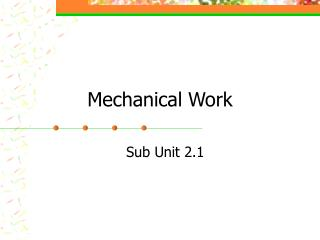# Mechanical Work - PowerPoint PPT PresentationDownload PresentationMechanical Work

Mechanical Work
Download Presentation## Mechanical Work

- - - - - - - - - - - - - - - - - - - - - - - - - - - E N D - - - - - - - - - - - - - - - - - - - - - - - - - - -
##### Presentation Transcript

1. Mechanical Work Sub Unit 2.1

2. Objectives • Define work done by a force or torque in a mechanical system. • Explain the relationship between work, force applied, and the distance an object moves. • Solve work problems, given force and distance information in English and SI units. • Explain how efficiency relates to input work and output work for a mechanical system. • Define radian measure of angles. • Explain the relationship between work, torque applied, and the angle (in radians) through which and object moves. • Solve work problems, given torque and angle information in English and SI units.

3. Work • Linear • When a force moves something a distance (like a pushing a car, moving a desk, lifting weights, etc.) • Rotational • When a torque causes rotational movement (like gears, pulleys, • wheels, etc.)

4. Work done by a force Work (W) = Force (F) x Distance (d) Work UnitsEnglishfoot • pounds (ft•lb) SInewton • meters (N•m) Force UnitsEnglishSI pounds newtons Distance UnitsEnglishSI feet meters = x 1 Newton meter (N•m) = 1 Joule (J)

5. Work • Uses magnitude of force and displacement (both are vectors) • Can be positive (if both force and displacement are in same direction) • Can be negative (if force and displacement are in opposite direction)

6. Change in Potential / Kinetic Energy? • Work equals change in energy • Like when the barbell gains gravitational potential energy due to the work done to lift it. • Or when braking, the kinetic energy of vehicle decreases due to the work done by the brakes.

7. output work Efficiency = input work Efficiency • Machines convert work input to useful work output. • Theoretically, “work in” equals “work out.” • Realistically, “work in” is always greater than “work out.” Why? – Losses To get percentage, multiply by 100%

8. Arc length  = r “Rotational Work” • Work done by a torque “” must be in radians Work (W) = torque () x angle moved ()  = F x l 1 rev = 360º = 2 rad

9. Summary • Mechanical systems use force and torque to cause desired movement and do useful work. • Work is done when a force or torque moves an object. Work is done only while the force or torque is applied in the direction of movement. • Work equals force times displacement or torque times angle. Work is measured in ft•lb or N•m (J= N•m). W=Fd; W=  • The displacement used to calculate work is the distance the object moves while the force is applied. • Efficiency describes how well a machine performs work. Efficiency is the ration of output work to input work (Eff = Wout / Win) • Angles can be measured in either radians or degrees. The radian is a dimensionless unit and is used in most calculations involving angles.